DG7YBN / 14 MHz / YBN 14-5wzs   Last Update Dec 28th, 2018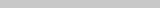.......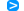Performance Data and GeometryPattern and VSWRPattern and VSWR over GroundValidation of Tapering YBN 14-5wzs   with Conventional Driven Element "Compact class 5 el. Yagi covering the 20 m band" The YBN 14-5wzs is a 28 ohms, short boom wideband beam covering the full 20 m band. Short boom means 13 meters. Which actually is short in wavelengths for a 5 element Yagi. Design date of issue: 2018.12.21 wzs = wide, non 50 ohms, short boom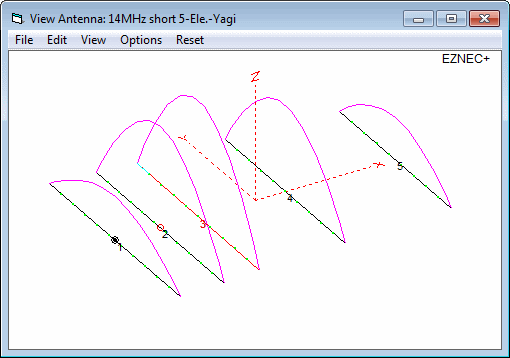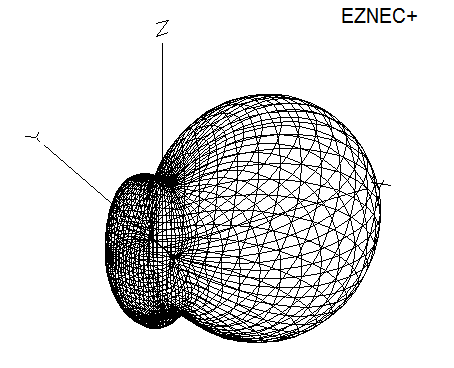Performance Data Specs: with 40 mm elements @ 14.2 MHz ``` Gain vs. isotr. Rad. 9.9 dBi Gain vs. Dipole 7.8 dBD -3 dB E-plane 55.2 deg. -3 dB H-plane 74.2 deg. F/B -24.3 dB F/R -17.0 dB Impedance 28 ohms VSWR Band Width 14.0 MHz = 1.15:1, 14.35 = 1.16:1 Mechan. Length 13,000 mm exclusive boom offsets for elem. plates Electr. Length 0.616 λ Stacking Dist. h-pol. top-to-bottom 17.5 m or 57.4 ft side-by-side 22.8 m or 74.7 ft ``` How many HF operators have been looking up this design since Dec. 2018?Geometry ... is available in request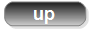Pattern and VSWR Plots Elevation and Azimuth plot at 14.2 MHz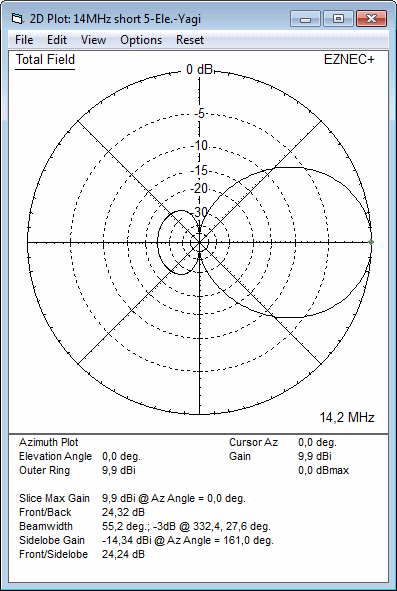RL and SWR plots - simulated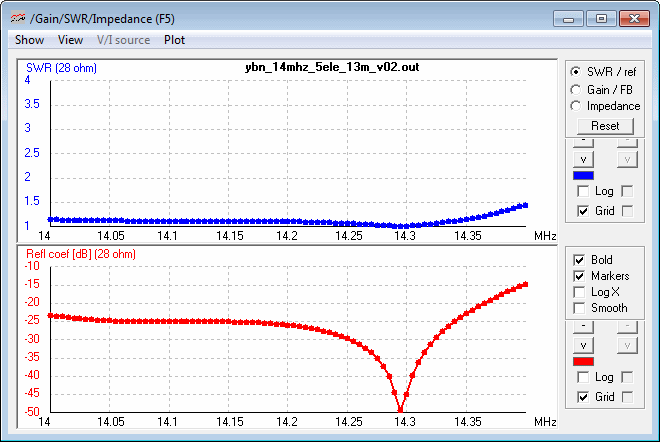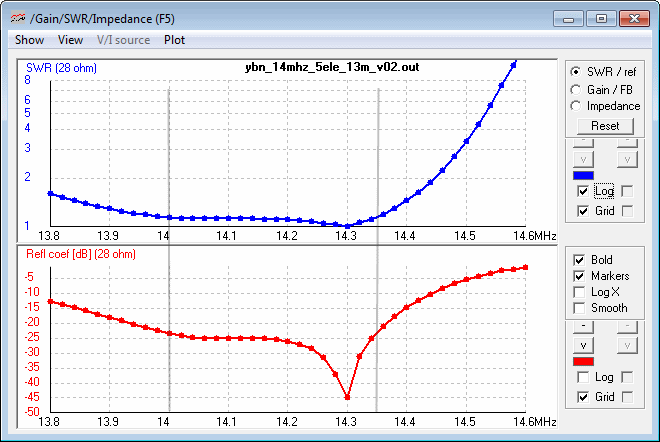Tapered Model Azimuth plot 14.0 - 14.35 MHz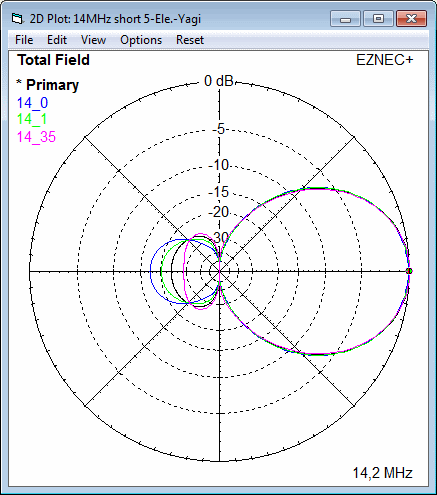14.00 MHz    F/B = 17.32 dB    Gain = 9.75 dBi 14.10 MHz    F/B = 20.34 dB    Gain = 9.89 dBi 14.20 MHz    F/B = 23.55 dB    Gain = 9.89 dBi 14.35 MHz    F/B = 28.75 dB    Gain = 9.87 dBiPattern and VSWR Plots over Ground Azimuth plot at 14.2 MHz at height of 18 m (perfect gnd assumed)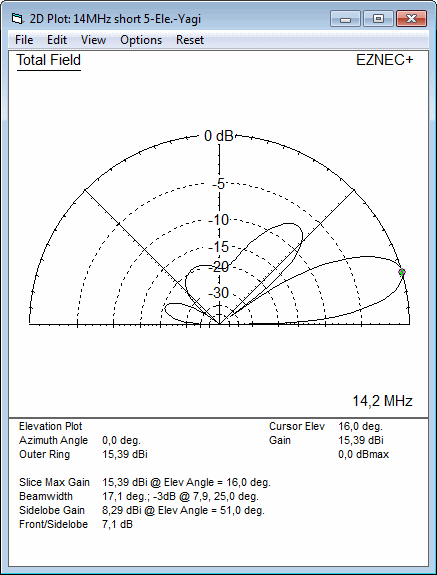Azimuth plot at 14.2 MHz at height of 24 m (perfect gnd assumed)VSWR plot at 14.2 MHz at height of 18 m (perfect gnd assumed)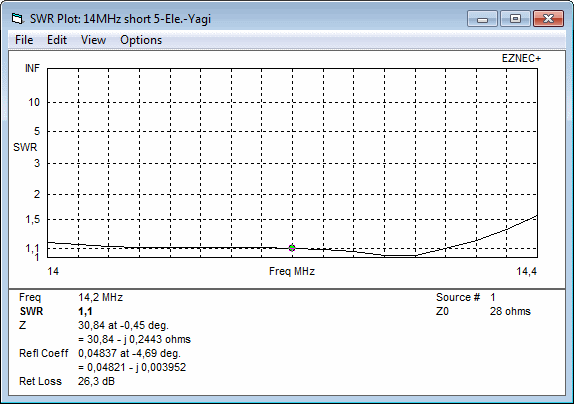VSWR and Return Loss plot at height of 18 m (perfect gnd assumed) 13.8 - 14.6 MHz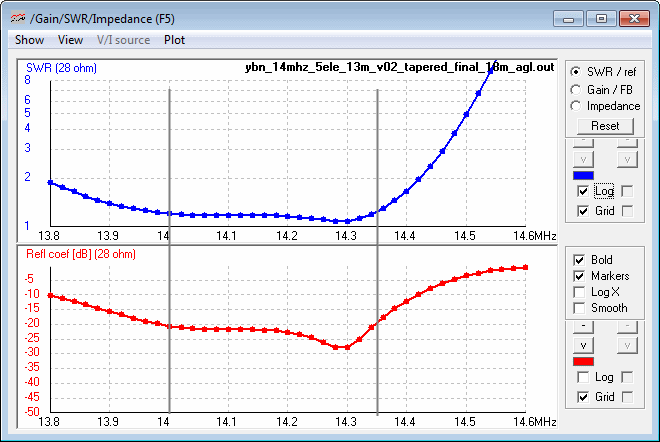VSWR and Return Loss plot at height of 24 m (perfect gnd assumed) 14.0 - 14.4 MHz Note: fine tuned > DE plus 15 mm per side = 2 x 5300 mm, D1 plus 10 mm per side = 2 x 5105 mm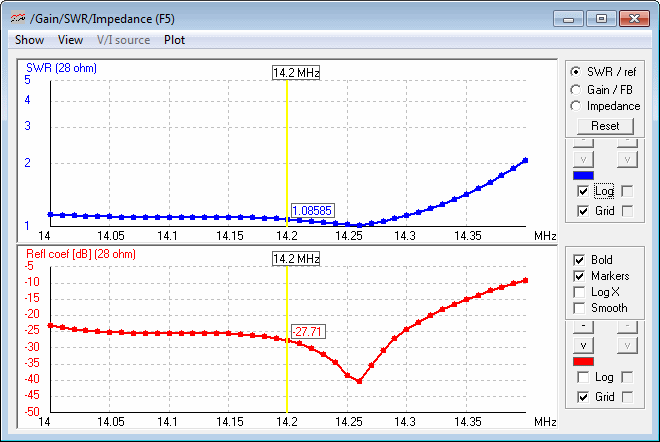Validation of Tapering Tapered Yagi: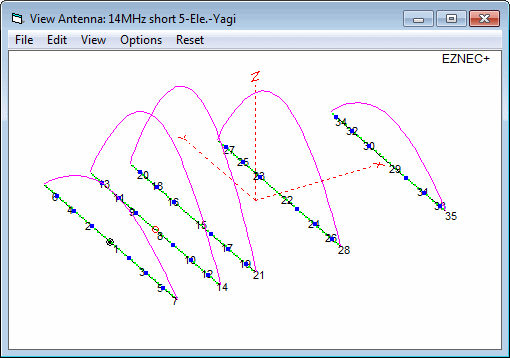Average Gain with Aluminium T-6061 for Elements = 0.988 = -0.05 dB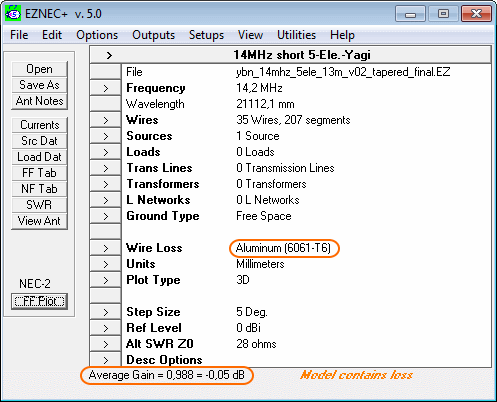Average Gain with zero wire losses = 1.000 = 0.00 dB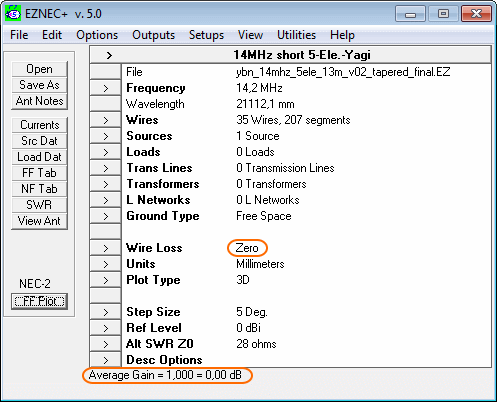73, Hartmut, DG7YBN